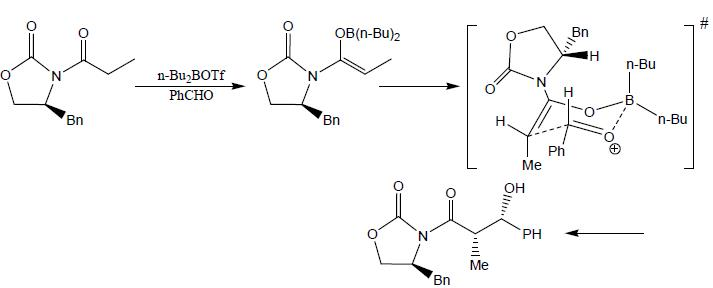Courses

# Reactive Inter & Reaction Mechanism

## 20 Questions MCQ Test GATE Chemistry Mock Test Series | Reactive Inter & Reaction Mechanism

Description
This mock test of Reactive Inter & Reaction Mechanism for Chemistry helps you for every Chemistry entrance exam. This contains 20 Multiple Choice Questions for Chemistry Reactive Inter & Reaction Mechanism (mcq) to study with solutions a complete question bank. The solved questions answers in this Reactive Inter & Reaction Mechanism quiz give you a good mix of easy questions and tough questions. Chemistry students definitely take this Reactive Inter & Reaction Mechanism exercise for a better result in the exam. You can find other Reactive Inter & Reaction Mechanism extra questions, long questions & short questions for Chemistry on EduRev as well by searching above.
QUESTION: 1

###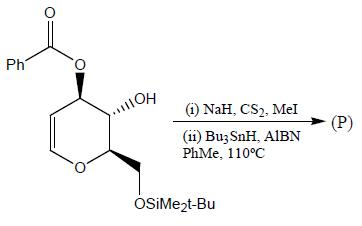Solution: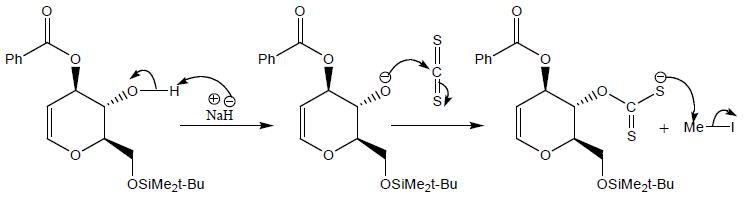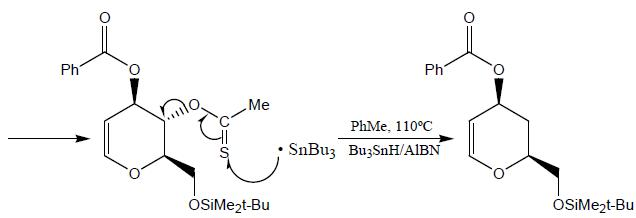QUESTION: 2

###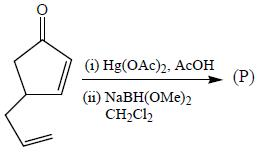Solution: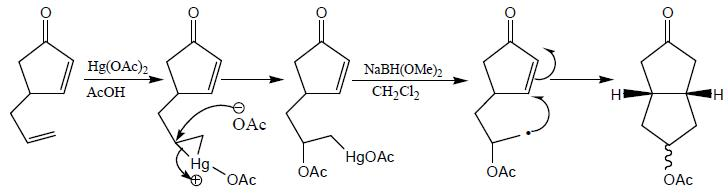QUESTION: 3

###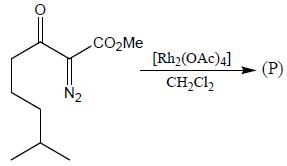Solution: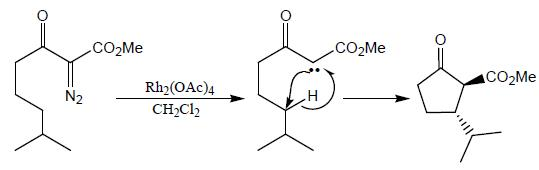QUESTION: 4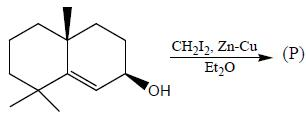Solution: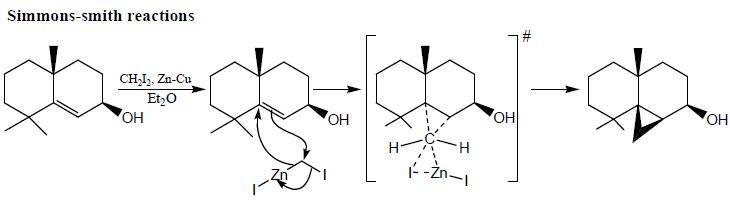This reaction is stereoselective, co-ordination of the oxygen atom to the sinc.

QUESTION: 5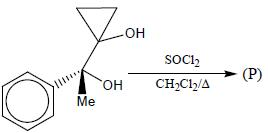The major product of the reaction is

Solution: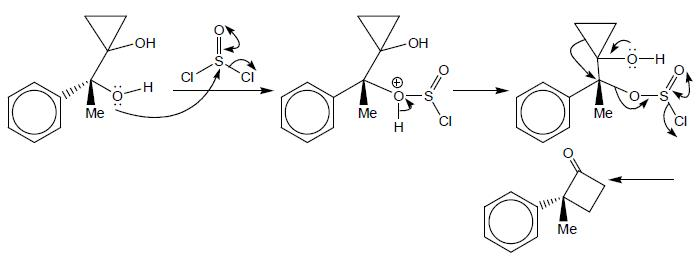QUESTION: 6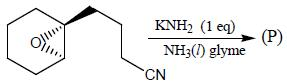Solution: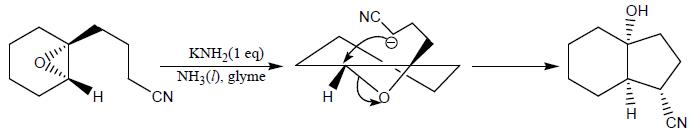*Answer can only contain numeric values
QUESTION: 7

How many monochlorinated products are possible including stereoisomer?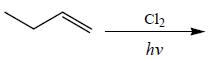Solution: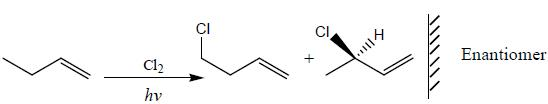QUESTION: 8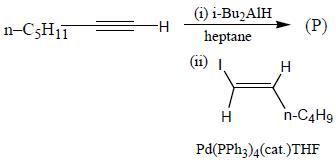Solution: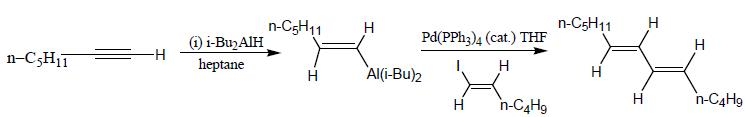QUESTION: 9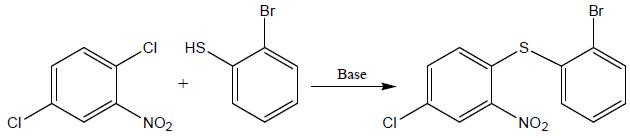Product formed in the above reaction, which of the following correct mechanism apply

Solution:

This reaction mechanism proceed through addition elimination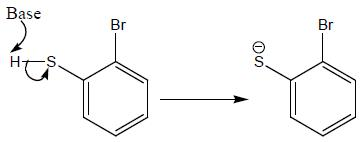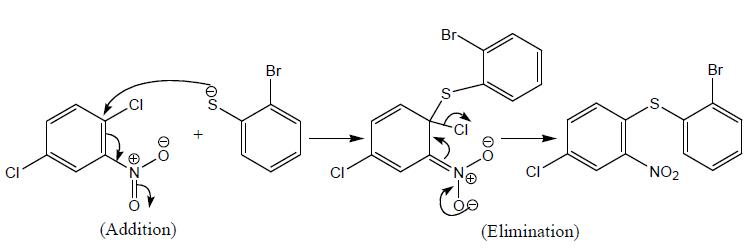QUESTION: 10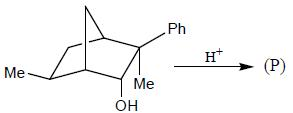Solution: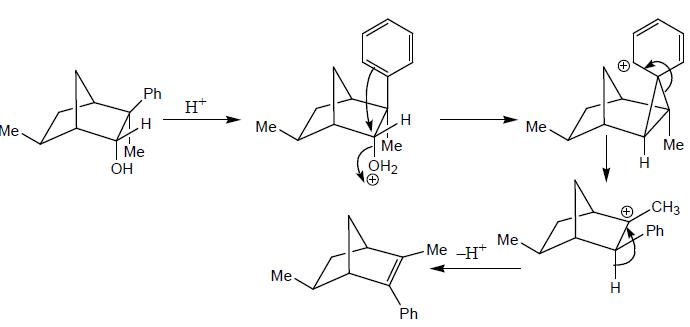QUESTION: 11

The reaction of the bromo compound shown below with sodium ethoxide gives predominantly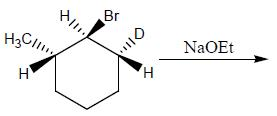Solution:

Chemical transformation involved in above chemical reaction can be illustrated as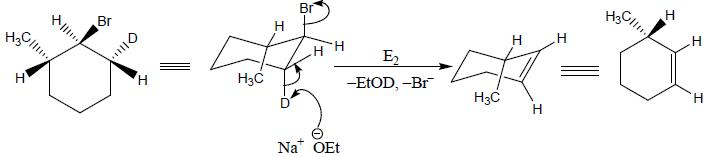QUESTION: 12

The major product obtained in the reaction below is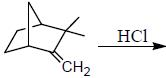Solution: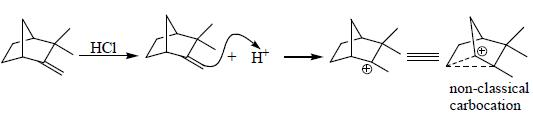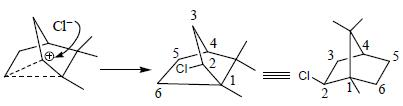*Answer can only contain numeric values
QUESTION: 13

The total number of compounds (shown below) that form phenylhydrazone derivatives under acidic conditions is ____________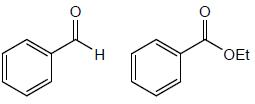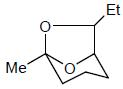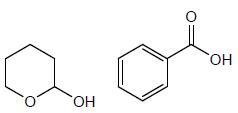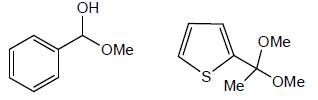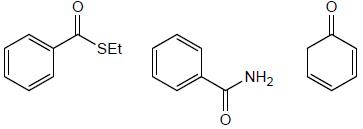Solution: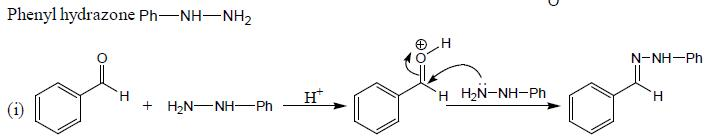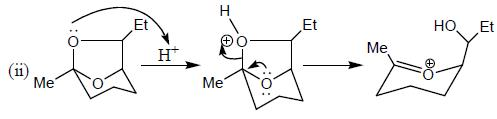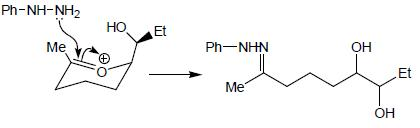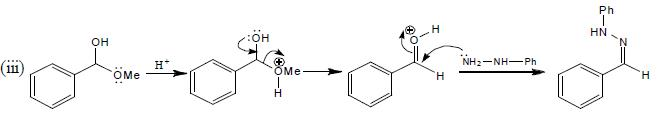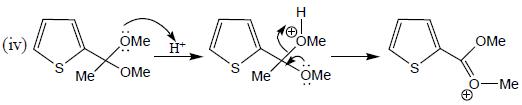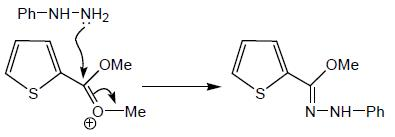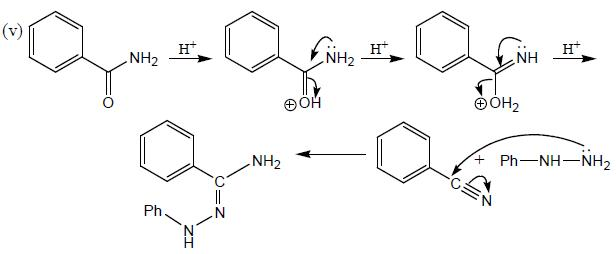From the above compound, five compound form phenylhydrozone derivative under acidic condition.

*Answer can only contain numeric values
QUESTION: 14

How many product will be formed the following reaction is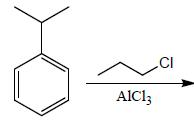Solution: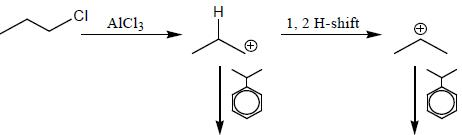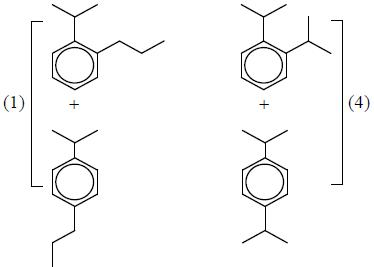QUESTION: 15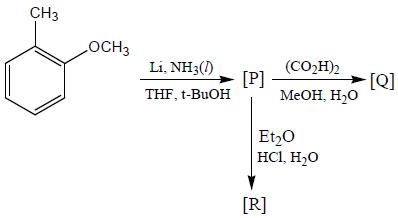Correct product (Q, R and P) in the above reactions are formed respectively

Solution: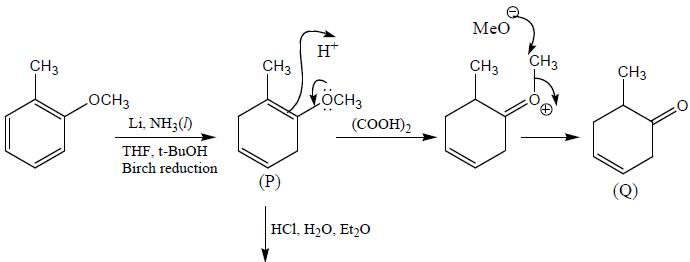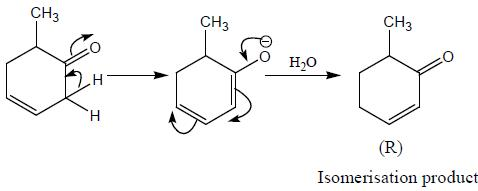QUESTION: 16

The major product (P) in the above reaction is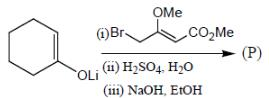Solution: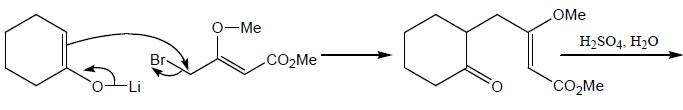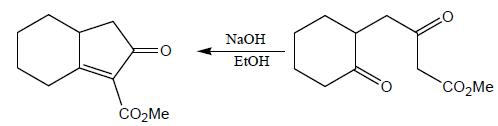QUESTION: 17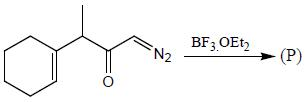Solution: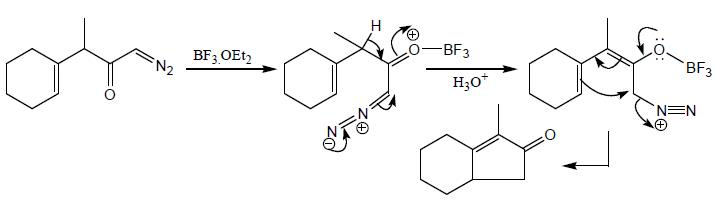QUESTION: 18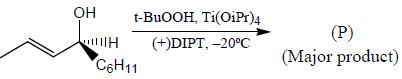Solution: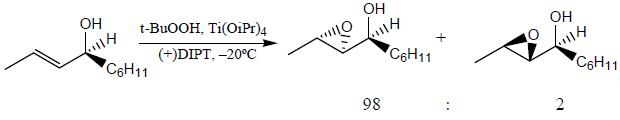Oxidation of the allylic alcohol using the chiral ligand (+) di-isopropyl tartrate, the (S) enantiomer reacts about a hundred times faster than the (R) enantiomers epoxide formed from less hindered side.

QUESTION: 19

Which of the following statement(s) is true about the reaction given below?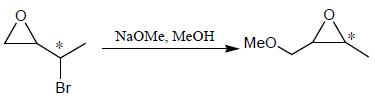(1) it involves a carbocation intermediate
(2) rearrangement is due to SN1 reaction mechanism.
(3) it proceeds via a concerted SN2 pathway
(4) it involves neighbouring group participation.

Solution: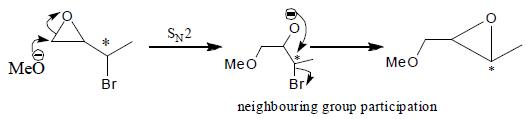From above mechanism:
If proceecs via a concerted SN2 pathway.
It involves neighbouring group participation.

QUESTION: 20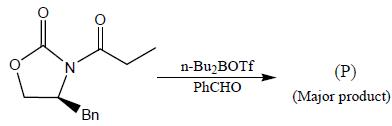Solution: